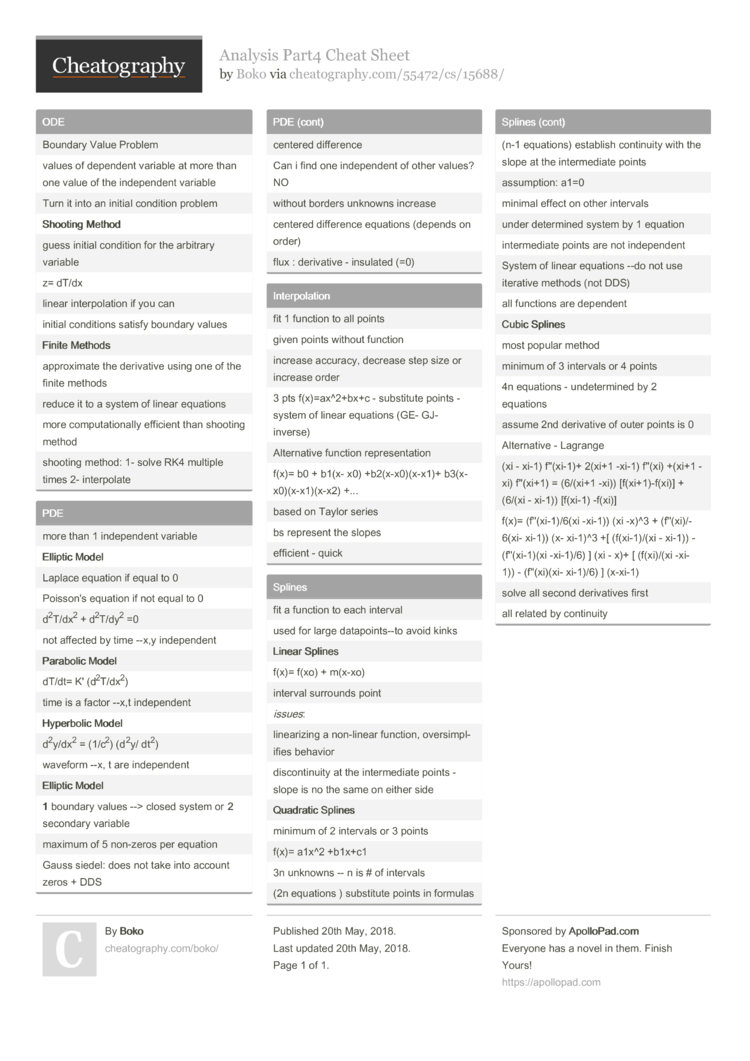Show Menu

# Analysis Part4 Cheat Sheet by Boko

### ODE

 Boundary Value Problem values of dependent variable at more than one value of the indepe­ndent variable Turn it into an initial condition problem Shooting Method guess initial condition for the arbitrary variable z= dT/dx linear interp­olation if you can initial conditions satisfy boundary values Finite Methods approx­imate the derivative using one of the finite methods reduce it to a system of linear equations more comput­ati­onally efficient than shooting method shooting method: 1- solve RK4 multiple times 2- interp­olate

### Interp­olation

 fit 1 function to all points given points without function increase accuracy, decrease step size or increase order 3 pts f(x)=a­x^2­+bx+c - substitute points - system of linear equations (GE- GJ- inverse) Altern­ative function repres­ent­ation f(x)= b0 + b1(x- x0) +b2(x-­x0)­(x-x1)+ b3(x-x­0)(­x-x­1)(­x-x2) +... based on Taylor series bs represent the slopes efficient - quick

### PDE

 more than 1 indepe­ndent variable Elliptic Model Laplace equation if equal to 0 Poisson's equation if not equal to 0 d2T/­dx2 + d2T/­dy2 =0 not affected by time --x,y indepe­ndent Para­bolic Model dT/dt= K' (d2T­/dx2) time is a factor --x,t indepe­ndent Hype­rbolic Model d2y/­dx2 = (1/c2) (d2y/ dt2) waveform --x, t are indepe­ndent Elliptic Model 1 boundary values --> closed system or 2 secondary variable maximum of 5 non-zeros per equation Gauss siedel: does not take into account zeros + DDS centered difference Can i find one indepe­ndent of other values? NO without borders unknowns increase centered difference equations (depends on order) flux : derivative - insulated (=0)

### Splines

 fit a function to each interval used for large datapo­int­s--to avoid kinks Linear Splines f(x)= f(xo) + m(x-xo) interval surrounds point issues: linear­izing a non-linear function, oversi­mpl­ifies behavior discon­tinuity at the interm­ediate points - slope is no the same on either side Quad­ratic Splines minimum of 2 intervals or 3 points f(x)= a1x^2 +b1x+c1 3n unknowns -- n is # of intervals (2n equations ) substitute points in formulas (n-1 equations) establish continuity with the slope at the interm­ediate points assump­tion: a1=0 minimal effect on other intervals under determined system by 1 equation interm­ediate points are not indepe­ndent System of linear equations --do not use iterative methods (not DDS) all functions are dependent Cubic Splines most popular method minimum of 3 intervals or 4 points 4n equations - undete­rmined by 2 equations assume 2nd derivative of outer points is 0 Altern­ative - Lagrange (xi - xi-1) f''(xi-1)+ 2(xi+1 -xi-1) f''(xi) +(xi+1 -xi) f''(xi+1) = (6/(xi+1 -xi)) [f(xi+­1)-­f(xi)] + (6/(xi - xi-1)) [f(xi-1) -f(xi)] f(x)= (f''(x­i-1­)/6(xi -xi-1)) (xi -x)^3 + (f''(x­i)/­6(xi- xi-1)) (x- xi-1)^3 +[ (f(xi-­1)/(xi - xi-1)) - (f''(x­i-1)(xi -xi-1)/6) ] (xi - x)+ [ (f(xi)/(xi -xi-1)) - (f''(x­i)(xi- xi-1)/6) ] (x-xi-1) solve all second deriva­tives first all related by continuity

## Download the Analysis Part4 Cheat Sheet1 Page
//media.cheatography.com/storage/thumb/boko_analysis-part4.750.jpg

PDF (recommended)

Alternative Downloads

## Comments

No comments yet. Add yours below!

## Add a Comment

Your Comment

Please enter your name.

Please enter your email address

Please enter your Comment.